# Matrix Blend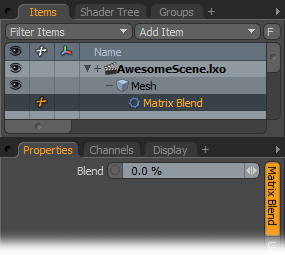You can use this Channel Modifier to blend between a pair of Rotation or Translation input matrices.

 Matrix A/Matrix B Specify the two matrices to be blended into these channels. These can be World or Local transform matrices from 3D items or the matrix output from other Channel Modifier items. For the best results, link channels with matrices of the same type. Blend Modo uses this blend amount to apply to the input matrices. With a value of 0%, Modo outputs the Matrix A matrix unaltered; with 100% Modo outputs the Matrix B matrix unaltered. For values between 0% and 100%, Modo interpolates the matrices between the two inputs. For Rotation matrices, Modo uses a quaternion interpolation, and for Translation matrices, Modo uses a linear interpolation. Matrix Output Modo passes the result of the interpolation to this channel. You can link this to a compatible matrix channel on a 3D item or another Channel Modifier.

# Matrix Compose

The Matrix Compose Channel Modifier multiplies two or more input matrices together and passes the resulting transform matrix.

 Matrix Inputs This channel accepts multiple input links from the matrix channels of 3D items or other Channel Modifier outputs. Modo multiples the matrices together in the order that you used when you linked them this channel. Matrix Output Modo outputs the composed matrix to this channel. You can link this into a compatible matrix channel on a 3D item or another Channel Modifier's input.

# Matrix Construct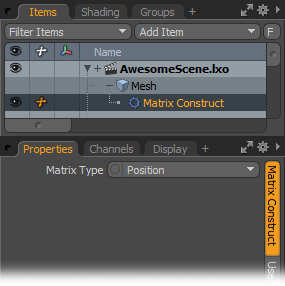The Matrix Construct modifier creates a 4x4 Transformation matrix from a vector.

 Input X/Input Y/Input Z Describes either the position or the scale of the resulting matrix. Matrix Type Specifies whether the output is a Position matrix or a Scale matrix. Output Modo passes the composed matrix to this channel.

# Matrix From Euler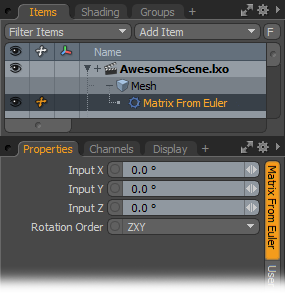The Matrix From Euler modifier extracts a vector that represents rotation angles in the X, Y and Z axes from a 4x4 Rotation matrix.

 Input X/Input Y/Input Z Describes rotation angles around the X, Y, and Z axes. Rotation Order Describes the rotation order of the three axes. With the default ZXY order, Modo calculates the final matrix by creating a matrix to represent the Z axis, then creating a matrix to represent the X axis, and finally creating a matrix to represent the Y axis. Modo then multiples the three matrices together to construct the final matrix. Output Outputs the resulting rotation matrix.

# Matrix To Euler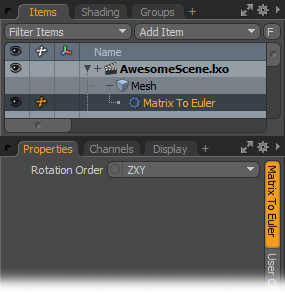The Matrix To Euler modifier extracts a vector that represents rotation angles in the X, Y, and Z axes from a 4x4 Rotation matrix.

 Input X/Input Y/Input Z Indicates the input rotation matrix from which to extract rotation angles. Rotation Order Describes the rotation order of the three axes. With the default ZXY order, Modo calculates the final matrix by creating a matrix to represent the Z axis, then creating a matrix to represent the X axis, and finally creating a matrix to represent the Y axis. Modo then uses the three matrices to construct the final matrix. Output X/Output Y/Output Z Describes rotation angles around the X, Y, and Z axes.

# Matrix Invert

The Matrix Invert modifier inverts the transforms stored in the input matrix. For example, if the input matrix stores rotations of 90, 45, and 180 degrees, the output matrix stores the opposite: -90, -45, and -180 degrees. It also outputs the inverse of position and scale, or a combination of all three.

This modifier can be extremely useful, as often it's desirable to perform complex 3D math operations at the center of the world. Multiplying a transform matrix by it's inverse transform matrix, results in an identity matrix. That is to say, a matrix that stores no position, rotation, and 100% scale. This allows you to easily transform an item back to its center position, perform a maths operation easily, and then transform it back by multiplying by the original transform.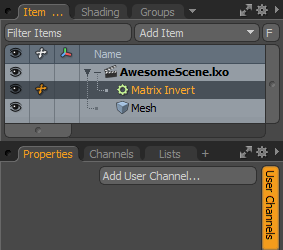Input The matrix to invert. Output The resulting inverse matrix.

# Matrix Transpose

The Matrix Transpose modifier inverts a 4x4 matrix across the main diagonal. Modo inverts any rotation stored in the matrix; therefore, for example, a rotation of 90 degrees in X becomes a rotation of -90 degrees.

 Input Indicates the matrix with the values to transpose. Output Indicates where Modo should pass the matrix with the transposed values.

# Matrix Vector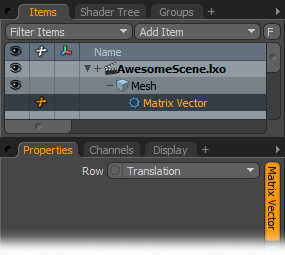You use this Channel Modifier to extract a normalized direction vector from a Rotation matrix or the translation row from a Translation matrix. Direction vectors in a Rotation matrix are the axes formed after the individual X, Y, and Z rotations have been performed. For example, given the World Rotation matrix of a 3D item, the Z Direction vector points in the direction the item is facing, and the Y axis points towards the top of the item.

 Matrix Input Links the matrix channel from which the vector is to be extracted into this channel. This can be a matrix channel from a 3D item or the output matrix from another Channel Modifier. Row This specifies whether to extract the X, Y, Z direction vector from a Rotation matrix or the translation from a Translation matrix. Output X/Output Y/Output Z Passes the vector extracted from the matrix to these three channels.

# Matrix Vector Multiply

The Matrix Vector Multiply modifier multiplies a transform matrix by a vector, and outputs the resulting vector. This essentially applies any transforms that are stored in the matrix to the vector. For example, if the vector stored a value of [1.0, 0.0, 0.0] and the matrix stored a position of 2m in X, then the resulting vector would be [3.0, 0.0, 0.0].

This modifier is really useful when used with rotation matrices. For example, if you have a ray that is fired from a point in space, by multiplying the ray vector by the rotation matrix, you can easily change the direction of the ray, rotating it by whatever value is stored in the matrix.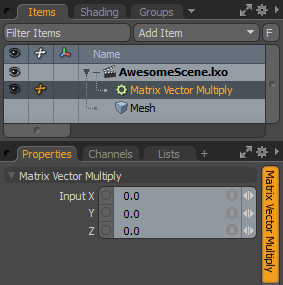Matrix The matrix to multiply the vector by. Input The vector to multiply by the matrix. Output The resulting vector.

# Twist Extractor

This channel modifier allows you to extract twist (roll), yaw, and pitch from a local matrix. The twist output can then be used to smooth deformations, for example, on the lower arm as the wrist is rotated or the lower leg as the foot is rotated.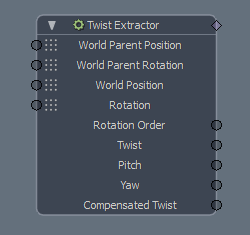To add a Twist Extractor node, in the Schematic, click Add... then under Channel Modifiers > Matrix, click Twist Extractor.

The node compares the twist of a joint to the Setup state, or another joint in the hierarchy.

Note:  For more information on Setup mode, see Binding and Setup Mode.

## Twist Extractor Channels on the Node

 World Parent Position The world position of the selected joint's parent joint. World Parent Rotation The world rotation of the selected joint's parent joint. World Position The selected joint's world position. Rotation The selected joint's rotation. Rotation Order The order of rotation for the matrix extraction. Twist The rotation of the selected joint around the longitudinal axis. Also called roll. Pitch The rotation of the selected joint around the transverse axis. Yaw The rotation of the selected joint around the vertical axis. Compensated Twist When a joint is rotated, the path it takes influences the orientation at the destination. For instance, the orientation differs when an arm is held forward compared to when it's held up. With a rotation order of Twist, Yaw, Pitch, Modo computes a compensated twist value to ensure that the orientation is the same at the destination.

## Twist Extractor Properties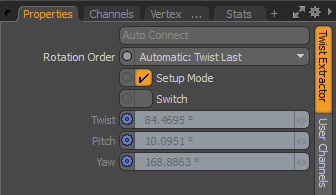Auto Connect When Setup Mode is disabled, you can use this to connect selected locators to the Twist Extractor node. With a locator and Twist Extractor selected, click this button to automatically set up the connections between the locator and the Twist Extractor node. Note:  When Setup Mode is enabled, Auto Connect is not available. Rotation Order The order the rotations are extracted from the matrix. In addition to a specific order of axes, you have the following options: • Automatic: Twist First - The default option. It extracts rotation starting with the twist axis and the others follow in direct rotation order. Z is usually the joint rotation axis, so the order is ZXY. • Automatic: Twist Last - It uses the twist axis as the last one and the others follow in direct rotation order. Usually, Z is the joint rotation axis, so the order is XYZ. Setup Mode When enabled (the default state), the Twist Extractor extracts the twist data from the difference between the current rotation compared to the Setup one. When disabled, the you can use Twist Extractor to get the twist values from a joint compared to its parent or another locator in the hierarchy. Switch The Twist Extractor calculates the twist in two different ways. In cases where the default calculation doesn't produce the expected result, enable Switch to calculate the twist using another method. Twist The rotation of the selected joint around the longitudinal axis. Also called roll. Pitch The rotation of the selected joint around the transverse axis. Yaw The rotation of the selected joint around the vertical axis.